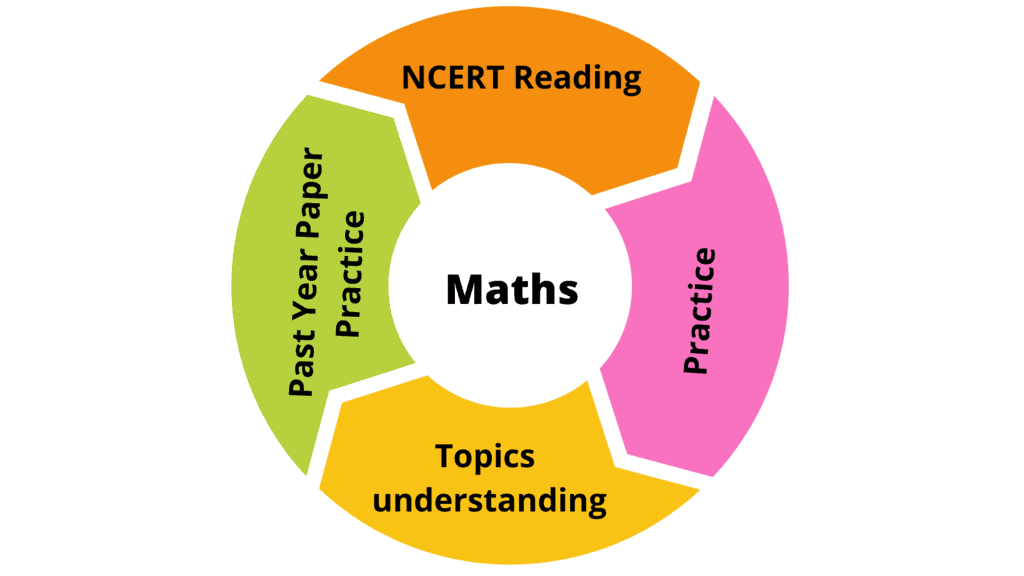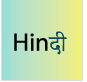How to prepare for Class 6 Mathematics: Tips & Tricks for Mathematics

# How to prepare for Class 6 Mathematics: Tips & Tricks for Mathematics - How To Study For Class 6

 Table of contents1. Read your NCERT Textbooks thoroughly2. Understand the Topics3. Practice4.  Practice Past Year PaperOne thing to be kept in mind all along the way for Maths preparation is that practice is the key here. The more you practice for every topic, the better understanding is achieved.

The main topics in Class 6 Maths are basic arithmetic, algebra, geometry and mensuration, probability, etc. These topics should be practiced as much as possible. The key to solve problems is to by-heart the formulas, understand the theorems, and practice as many times as possible. Maths can become your favorite subject with practice and dedication.Mathematics Course Class 6- Notes, Questions, Videos & MCQs

Includes 68 docs, 222 videos & 26 testsTips & Tricks for Mathematics :

Study from the Mathematics NCERT Textbook and practice all the solved examples and exercise questions thoroughly before moving forward to extra practice from additional books. Refer to NCERT Solutions in case of any doubt.

### NCERT Textbooks and Solutions

Chapter 1 - Knowing Our Numbers

Chapter 2 - Whole Numbers

Chapter 3 - Playing with Numbers

Chapter 4 - Basic Geometrical Ideas

Chapter 5 - Understanding Elementary Shapes

Chapter 6 - Integers

Chapter 7 - Fractions

Chapter 8 - Decimals

Chapter 9 - Data Handling

Chapter 10 - Mensuration

Chapter 11 - Algebra

Chapter 12 - Ratio and Proportion

Chapter 13 - Symmetry

Chapter 14 - Practical Geometry

## 2. Understand the Topics

Develop a good understanding of topics. It will help you with those challenging questions or derive formulae in the rare chance you forget. This can be done by watching videos for various topics that will help you develop an interest in the topic.

## 3. Practice

• Practice, above all. The range of questions in class 6 is limited. With substantial practice, most question types will be internalized, and you won’t have to think for most questions.
• Don’t just read or mug up the topics but practice them. This is the most important thing which you need to keep in mind as reading maths does not help you. Instead, make a routine and give some time for practice.
• Understand all the concepts in as much depth as you can. And then solve different varieties of questions and problems. Attempt various topic-wise and chapter-wise tests. Also, practice mre with sample papers and previous year papers. Use your knowledge to solve some real life questions as well.

## 4.  Practice Past Year Paper

Give more importance to the topics which have high weightage. Solve the previous year exam papers which will increase your confidence and give you a pre-exam feel. It also helps to brush up all your concepts before the exam.

### Important Topics and Preparation tips for Each Chapter

Chapter 1: Knowing our numbers

This is an introduction to the number system. Students get to understand and appreciate the meaning of the words thousand, lakhs, millions etc, and are expected to know how to write big denominations of numbers and count them in this chapter in 6th class maths. They learn the Indian system of counting and the International system of counting.

Chapter 2: Whole numbers

• This introduces students to natural, whole, non-decimal numbers. It introduces the concepts of predecessors and successors in the number system.

Chapter 3: Playing with numbers

• This is an introduction for students to the concept of factors of numbers, multiples of numbers etc.

Chapter 4: Basic geometrical ideas

• This introduces students to the concepts of point, line, rays, segments etc.

Chapter 5: Understanding elementary shapes

• This is an introduction to basic shapes like circle, triangles, rectangles, squares etc.

Chapter 6: Integers

• This chapter is about conceptualizing the number line and the positions of the numbers on the same. It enables students to have a vision about which number lies where which is more or which is less etc. This is an important chapter in 6th class maths.

Chapter 7: Fractions

• This chapter introduces the concepts of fractions as parts of a whole.

Chapter 8: Decimals

• This is an introduction to the concept of point and decimals, and their relationship to fractions.

Chapter 9: Data handling

• This shows students how to arrange numbers or items in a list according to a specified order using tally marks. It is a prerequisite to study statistics.

Chapter 10: Mensuration

• Teaches concepts of perimeter and areas of simple geometric figures.

Chapter 11: Ratio and proportion

• Building on the previous concepts of fractions, this chapter prepares the student to see ratios and proportions as relationships of parts with respect to the whole.

Chapter 12: Algebra

• This introduces students to the concepts of algebra; finding out the value of an unknown quantity using what information is known, or assessing the relationship between unknown variables using known variables.

Chapter 13: Symmetry

• This chapter builds on the conception and understanding of geometrical figures and shapes to introduce the concept of symmetry to students. Students are given incomplete figures and are expected to make them symmetric, or identify how given figures are symmetric or not, etc.

Chapter 14: Practical geometry

• This chapter is an introduction to how to draw basic geometric shapes using the geometric equipment. Students are given specific instructions and are expected to follow the same to draw the figures.
For Example: – Draw two circles of radii 2.5 cm and 4 cm which have a common centre. Students are expected to measure these radii on their scales and use their compasses to draw these circles to complete the exercise.

###Social Science Studies (SST) Class 6 (VI) - CBSE, NCERT

Includes 103 docs, 66 videos & 58 testsScience for Class 6 (VI) - CBSE & NCERT Curriculum

Includes 90 docs, 90 videos & 32 testsEnglish for Class 6 (VI) - CBSE and NCERT Curriculum

Includes 48 docsHindi (Vasant) for Class 6 (VI) - CBSE and NCERT Curriculum

Includes 46 docs & 30 videosEnglish (A Pact with the Sun) for Class 6 (VI)

Includes 20 docs & 53 videos

The document How to prepare for Class 6 Mathematics: Tips & Tricks for Mathematics | How To Study For Class 6 is a part of the Class 6 Course How To Study For Class 6.
All you need of Class 6 at this link: Class 6

12 docs

## FAQs on How to prepare for Class 6 Mathematics: Tips & Tricks for Mathematics - How To Study For Class 6

 1. How can I effectively prepare for my Class 6 Mathematics exam?2. How can reading NCERT textbooks help in preparing for my Class 6 Mathematics exam?Ans. Reading NCERT textbooks is crucial for preparing for your Class 6 Mathematics exam because: 1. Comprehensive Content: NCERT textbooks cover all the topics and concepts prescribed in the Class 6 Mathematics syllabus. They provide a comprehensive understanding of the subject. 2. Easy Language: The language used in NCERT textbooks is simple and easy to understand, making it easier for students to grasp the concepts. 3. In-depth Explanation: NCERT textbooks provide in-depth explanations of various mathematical concepts, ensuring that students have a strong foundation in the subject. 4. Exam-oriented Approach: The content in NCERT textbooks is designed keeping in mind the exam pattern and requirements. They provide ample practice exercises and examples to help students prepare effectively for their exams. 5. Credibility: NCERT textbooks are widely recognized and recommended by education boards. They are designed by experts in the field and are considered reliable sources of information.
 3. How can solving past year papers help in my Class 6 Mathematics exam preparation?Ans. Solving past year papers can be beneficial for your Class 6 Mathematics exam preparation in the following ways: 1. Familiarity with Exam Pattern: By solving past year papers, you become familiar with the exam pattern, question types, and marking scheme. This helps you understand what to expect in the actual exam. 2. Time Management: Practicing past year papers helps you develop effective time management skills. You get an idea of how much time to allocate to each question, ensuring that you can complete the paper within the given time limit. 3. Identifying Weak Areas: By analyzing your performance in past year papers, you can identify your weak areas and focus on improving them. This helps you allocate your study time more efficiently. 4. Confidence Building: Solving past year papers gives you confidence as you become more familiar with the exam format and the types of questions asked. This boosts your confidence and reduces exam anxiety. 5. Practicing Exam-like Conditions: Solving past year papers under exam-like conditions helps you get accustomed to the exam environment and reduces the chances of being overwhelmed during the actual exam.
 4. Is it necessary to understand the topics thoroughly for Class 6 Mathematics exam preparation?Ans. Yes, it is crucial to understand the topics thoroughly for Class 6 Mathematics exam preparation. Here's why: 1. Strong Foundation: Understanding the topics thoroughly ensures a strong foundation in mathematics. This helps in building advanced mathematical concepts in higher classes. 2. Problem-solving Skills: Thorough understanding of topics allows you to develop better problem-solving skills. It helps you apply the concepts learned to solve various mathematical problems effectively. 3. Conceptual Clarity: When you understand the topics thoroughly, you have a clear understanding of the underlying concepts. This clarity helps in retaining information and applying it in different scenarios. 4. Higher Order Thinking: Thorough understanding of topics enables you to think critically and analytically. It helps you develop higher order thinking skills, which are essential for solving complex mathematical problems. 5. Confidence Boost: When you understand the topics thoroughly, you gain confidence in your ability to tackle any question related to those topics. This confidence reflects in your exam performance.
 5. How can practicing regularly help in my Class 6 Mathematics exam preparation?Ans. Regular practice is essential for effective preparation for your Class 6 Mathematics exam. Here's why: 1. Reinforcement of Concepts: Regular practice helps reinforce the concepts learned in class. This repetition improves your understanding and retention of mathematical concepts. 2. Skill Development: Regular practice enhances your problem-solving skills. It helps you become faster and more accurate in solving mathematical problems. 3. Time Management: Practicing regularly helps you manage your time better. With regular practice, you become more efficient in solving problems within the given time limit. 4. Confidence Building: Regular practice boosts your confidence as you become more comfortable with the subject. It reduces exam anxiety and helps you perform better in the actual exam. 5. Identification of Weak Areas: Regular practice allows you to identify your weak areas and focus on improving them. It helps you allocate your study time more effectively, targeting areas that need more attention.

## How To Study For Class 6

12 docsExplore Courses for Class 6 examSignup to see your scores go up within 7 days! Learn & Practice with 1000+ FREE Notes, Videos & Tests.
10M+ students study on EduRev
Track your progress, build streaks, highlight & save important lessons and more!
Related Searches

,

,

,

,

,

,

,

,

,

,

,

,

,

,

,

,

,

,

,

,

,

;